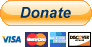# easy-memoize

## Usage no npm install needed!

``````<script type="module">
import easyMemoize from 'https://cdn.skypack.dev/easy-memoize';
</script>``````

# easy-memoize

Memoization is a simple thing and should be made easy to implement. The library `easy-memoize` will help you with it. For an enhanced documentation please visit the wiki pages.

# Usage

The usage of this library is as easy as 1, 2, 3. Just wrap it around your called function and pass in the arguments, like you normally would.

``````import easyMemo from "easy-memoize";

/* ... */

// On first call calculates the result of 1 * 2, if this code ever gets called again, the cached result will be returned.
easyMemo((a, b) => a * b, [])(1, 2); // returns: 2

// It returns the same object if the dependency is the same ( === safe)
easyMemo((value) => ({ value, randomProp: "abc" }), [])("R3DST0RM"); // returns: { value: "R3DST0RM", randomProp: "abc" }
``````

## Memoize Signature

The memoize function receives two input params, the function to memoize as well as an array of dependencies. When those dependencies change, the function will be executed again otherwise a cached result will be returned.

E.g: `easyMemo(() => { return anotherFunction() }, [anotherFunction])`

If `anotherFunction` changes, the memoized function will be executed again.

## Memoize Limits

The standard cache size of one function is at 10. Means, 10 results will be cached. If a function exceeds this limit, the oldest results will be removed.

Using `overrideMaxCacheSize(num: Number)` allows you to override this default behavior.

# How it works

The memoization is done by storing the function and its arguments. If one of it changes, the function will be executed again.

Let's assume there is a function called: `heavyCalculation` with the following implementation:

``````const fibonacci = (num) => num <= 1 ? 1 : fibonacci(num - 1) + fibonacci(num - 2);

const heavyCalculation = () => fibonacci(40);
``````

The bet is, you would not want it to run again and again everytime the result is needed, just once, until something changes.

Using easy-memoize, this would be achieved wrapping `heavyCalculation` with the memoize function:

``````const easyHeavyCalculation = easyMemo(heavyCalculation, []) // returns a new memoized function

// By running easyHeavyCalculation(); a cached value will be returned if it gets executed a second time
console.log(easyHeavyCalculation());
console.log(easyHeavyCalculation()); // returns cached value
``````

# Motivation

As motivation served the `useCallback` function from React. Where it is possible to memoize a function call based on it's dependencies.

Therefore this library strives to be as efficient as possible while maintain the easiness of `useCallback`.

# Contribute

Your contribution is highly wanted. If there is a feature or issue you want to work an feel free to submit a PR.

# Browser Support

We care about browser support. Therefore this library has support for Internet Explorer 11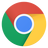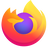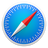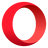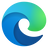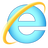Latest ✔ Latest ✔ Latest ✔ Latest ✔ Latest ✔ 11 ✔

# Donate

If you like this library and would like to support this work feel free to donate here: